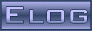DRS4 Forum
 DRS4 Discussion Forum Not logged inMessage ID: 773     Entry time: Fri Sep 13 15:27:41 2019
 Author: Arseny Rybnikov Subject: Scaler / How to modify the firmware to change the scaler integration time

Hello,

We want to use the inner DRS4 counter(scaler) within more than the 100ms integration time. We guess that we need to modify the original firmware around this point:

-- Reference clock used for frequency counter

proc_1hzclk: process(I_RESET, I_CLK33)
begin
if (I_RESET = '1') then
drs_1hz_counter(31 downto 0)          <= (others => '0');
drs_1hz_clock                         <= '0';
scaler_reset                          <= (others => '1');
scaler_ff_reset                       <= (others => '1');
elsif rising_edge(I_CLK33) then
drs_1hz_counter                       <= drs_1hz_counter - 1; -- count down
scaler_reset                          <= (others => '0');
scaler_ff_reset                       <= (others => '0');

-- toggle refclk if timer expires
if (drs_1hz_counter(drs_1hz_counter'high) = '1') then
drs_1hz_clock                       <= not drs_1hz_clock;
drs_1hz_counter(31 downto 0)        <= X"0016E35F";     -- 1499999, I_CLK33 is actually a 30 MHz clock

scaler_ff_reset                     <= (others => '1'); -- reset scaler_ff once every 100ms cycle
loop_scaler_reset : for i in 0 to 5 loop
if (scaler_ff(i) = '0') then                          -- no activity since last cycle?
scaler_reset(i)                <= '1';             -- force clear scaler register
end if;
end loop;

if (scaler_ff(0) = '0') then                            -- no activity since last cycle?
scaler_reset(0)                   <= '1';             -- force clear scaler register
end if;

end if;
end if;
end process;

Could you please tell us how to modify the firmware to increse the time up to 5 seconds for instance?

Thanks in advance, Arseny

ELOG V3.1.4-bcd7b50